# Math Olympiad Test: Statistics- 3 - Class 10

Test Description

## 10 Questions MCQ Test Olympiad Preparation for Class 10 - Math Olympiad Test: Statistics- 3

Math Olympiad Test: Statistics- 3 for Class 10 2023 is part of Olympiad Preparation for Class 10 preparation. The Math Olympiad Test: Statistics- 3 questions and answers have been prepared according to the Class 10 exam syllabus.The Math Olympiad Test: Statistics- 3 MCQs are made for Class 10 2023 Exam. Find important definitions, questions, notes, meanings, examples, exercises, MCQs and online tests for Math Olympiad Test: Statistics- 3 below.
 1 Crore+ students have signed up on EduRev. Have you?
Math Olympiad Test: Statistics- 3 - Question 1

### The mean of 1, 3, 4, 5, 7, 4 is m. The numbers 3, 2, 2, 4, 3, 3, p have mean m – 1 and median q. Then, p + q =

Detailed Solution for Math Olympiad Test: Statistics- 3 - Question 1

Mean of 1,3, 4, 5, 7 and 4 is m.
⇒ 1 + 3 + 4 + 5 + 7 + 4 / 6 = m
⇒ m = 4
Now, mean of 3, 2, 2, 4, 3, 3 and p is m - 1
3 + 2 + 2 + 4 + 3 + 3 + p / 7 = 3
⇒ 17 + p = 21 ⇒ p = 4
Arranging 3, 2, 2, 4, 3, 3, and 4 in ascending order, we get 2, 2, 3, 3, 3, 4, 4
∴ Median (q) =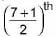term = 4th term = 3
⇒ p + q= 4 + 3 = 7

Math Olympiad Test: Statistics- 3 - Question 2

### Find the mode for the following data.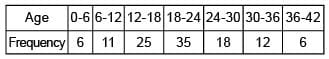Detailed Solution for Math Olympiad Test: Statistics- 3 - Question 2

Since, maximum class frequency is 35, so the mode class is 18 - 24.

Now, Mode =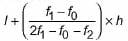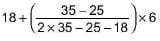= 1 8 + 2.22 = 20.22

Math Olympiad Test: Statistics- 3 - Question 3

### Which of the following is not a measure of central tendency?

Detailed Solution for Math Olympiad Test: Statistics- 3 - Question 3

Mean, median and mode are the measures of central tendency.

Math Olympiad Test: Statistics- 3 - Question 4

The median of a given frequency distribution is found graphically with the help of  ____.

Detailed Solution for Math Olympiad Test: Statistics- 3 - Question 4

Ogive or cumulative frequency curve is used to find the median.

Math Olympiad Test: Statistics- 3 - Question 5

The mean of 9 observations is 36. If the mean of the first 5 observations is 32 and that of the last 5 observations is 39, then the fifth observation is _____.

Detailed Solution for Math Olympiad Test: Statistics- 3 - Question 5

36 x 9 = x1 + x2 + x3 + . . . + x9 ... (i)
5 x 32 = x1 + x2 + x3 + x4 + x5 ,..(ii)
5 x 39 = x5 + x6 + x7 + x8 + x9 ...(iii)

we get 355 = x 1 + x2 + x 3 + x4 + x 5 + ... + x9 + X5

Using (i), we get 355 - 36 x 9 = x5

x5 = 31

Math Olympiad Test: Statistics- 3 - Question 6

The average monthly income (in ₹) of certain agricultural workers is S and that of other workers is T. The number of agricultural workers are 11 times that of other workers. Then the average monthly income (in `)of all the workers is ____.

Detailed Solution for Math Olympiad Test: Statistics- 3 - Question 6

Let the number of agricultural and other workers be n1 and n2.
∴ n= 11n2    ......(i)
Sum of monthly income of agricultural workers = Sn1
Sum of monthly income of other workers = Tn2
Sum of monthly income of all workers = Sn1  + Tn2
∴ Average monthly income of all workers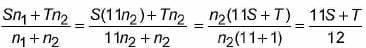Math Olympiad Test: Statistics- 3 - Question 7

The mean weight of a class of 34 students is 46.5 kg. If the weight of the teacher is included, the mean rises by 500g. Then the weight of the teacher is _____.

Detailed Solution for Math Olympiad Test: Statistics- 3 - Question 7

It is given that,
Mean weight of 34 students = 46.5kg
So the total weight = 34 (46.5) = 1581kg
We know that,
Mean weight of 34 students and teacher = 46.5 + 0.5 = 47kg
So the total weight of 34 students and teacher = 47 (35) = 1645kg
The weight of teacher = Weight of 34 students and teacher – total weight
By substituting the values,
Weight of the teacher = 1645 – 1581 = 64kg
Therefore, the weight of the teacher is 64kg.

Math Olympiad Test: Statistics- 3 - Question 8

The average temperature of the town in the first four days of a month was 58 degree. The average for the second, third, fourth and fifth days was 60 degree. If the temperatures of the first and fifth days were in the ratio 7 : 8, then what is the temperature on the fifth day?

Detailed Solution for Math Olympiad Test: Statistics- 3 - Question 8

Let the temperatures on first, second, third, fourth and fifth day be x1, x2, x3, x4 and x5, respectively
Sum of temperatures of Ifirst four days = 58 * 4

i.e ., x1 + x2 + x3 + x4 = 232 ...(i)

and sum of temperatures on second, third, fourth and fifth day = 60 x 4

i.e., x2 + x3 + x4 + x5- = 240 ...(ii) Subtracting (i) from (ii), we get x5- x1 = 8 ...(iii)

Also, temperature of first and fifth day were in the ratio 7 : 8. Let the temperatures be 7 k and 8k respectively
From (iii), we have 8k - 7k = 8

⇒ k = 8

Temperature of fifth day = 8 x 8 = 64 degree.

Math Olympiad Test: Statistics- 3 - Question 9

The median of the following data is 525. Find the missing frequencies, if it is given that there are 100 observations in the data.

Detailed Solution for Math Olympiad Test: Statistics- 3 - Question 9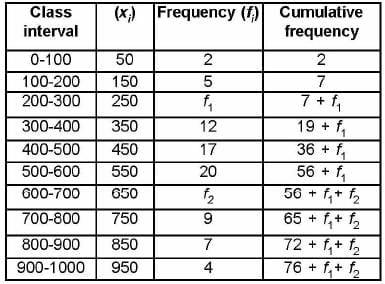We have given n = 100

⇒ 76 + f1 + f2 = 100

⇒  f1 + f2 = 24                 ....(i)

Since median is 525, so median class is 500 - 600.

Median =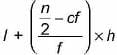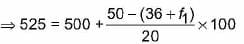⇒ 25 = (14 - f1) x 5 ⇒ 25 = 70 - 5f1

⇒ 5f1, = 45

⇒ f1 = 9

From (i), 9 + f2 = 24

⇒ f2 = 24 - 9 = 15

Math Olympiad Test: Statistics- 3 - Question 10

In an examination of 675 candidates of maximum marks 100 the examiner supplied the following information.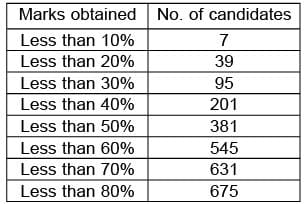Calculate median and mode respectively of the percentage marks obtained.

Detailed Solution for Math Olympiad Test: Statistics- 3 - Question 10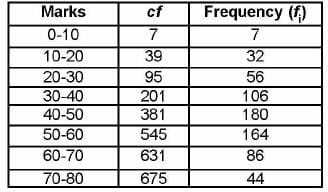Here, n = 675

⇒ n/2 = 337.5

So, Median class 40 - 50.

Median =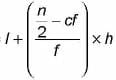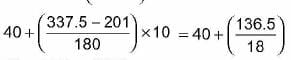= 40 + 7.58 = 47.58

Now, maximum frequency is 180.
So, modal class is 40 - 50.

Mode =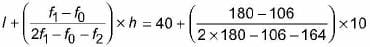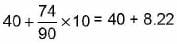= 48.22

## Olympiad Preparation for Class 10

11 videos|36 docs|201 tests